# Statistics with SciPy and Statsmodels

## Libraries for statistics

SciPy is a Python package with a large number of functions for numerical computing. It also contains statistical functions, but only for basic statistical tests (t-tests etc.). More advanced statistical tests are provided by Statsmodels. Statsmodels is powerful, but not very user-friendly; therefore, the tutorial below shows examples of several commonly used statistical tests.

All datasets used below are taken from the example data included with JASP, with the exception of the Zhou et al. (2020) dataset used for the Repeated Measures ANOVA.

## T-tests

### Independent-samples t-test

Consider this dataset from Matzke et al. (2015). In this dataset, participants performed a memory task in which they recalled a list of words. During the retention interval, one group of participants looked at a central fixation dot on a display. Another group of participants continuously made horizontal eye movements, which is believed by some to improve memory.

You can use the `ttest_ind()` function from `scipy.stats` to test whether memory performance (`CriticalRecall`) was higher for the horizontal-eye-movement group as compared to the fixation group. (There is a significant difference, but it goes in the opposite direction, such that the fixation group performed best.)

```from datamatrix import io
from scipy.stats import ttest_ind

dm_horizontal, dm_fixation = ops.split(dm.Condition, 'Horizontal', 'Fixation')
t, p = ttest_ind(dm_horizontal.CriticalRecall, dm_fixation.CriticalRecall)
print('t = {:.4f}, p = {:.4f}'.format(t, p))
```

Output:

```t = -2.8453, p = 0.0066
```

It's always helpful to visualize the results:

```from matplotlib import pyplot as plt
import seaborn as sns

sns.barplot(x='Condition', y='CriticalRecall', data=dm)
plt.xlabel('Condition')
plt.ylabel('Memory performance')
plt.show()
```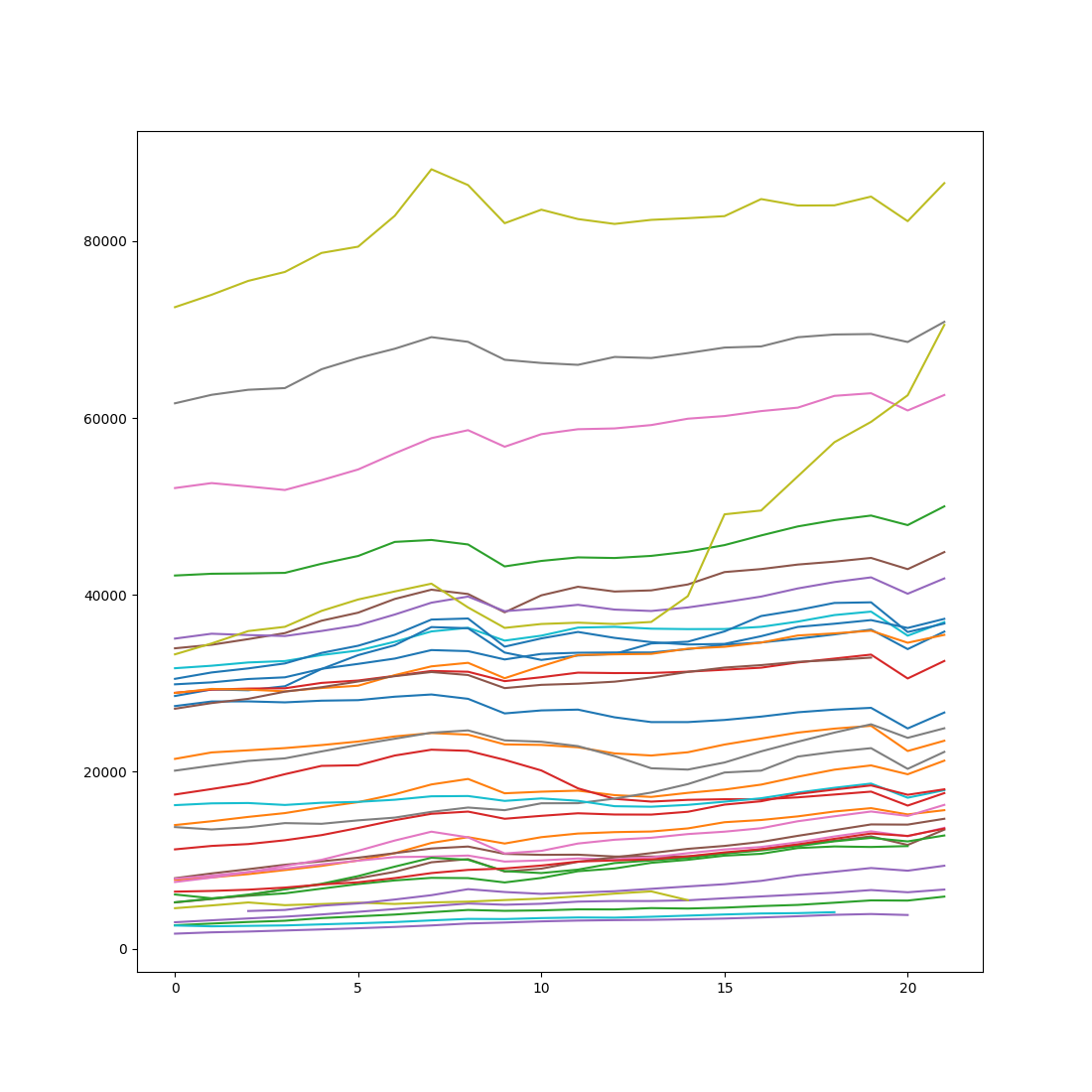### Paired-samples t-test

Consider this dataset from Moore, McCabe, & Craig. Here, aggressive behavior from people suffering from dementia was measured during full moon and another phase of the lunar cycle. Each participant was measured at both phases, i.e. this was a within-subject design.

You can use the `ttest_rel()` function to test whether aggression differed between the full moon and the other lunar phase. (Interestingly, it did.)

```from datamatrix import io
from scipy.stats import ttest_rel

t, p = ttest_rel(dm.Moon, dm.Other)
print('t = {:.4f}, p = {:.4f}'.format(t, p))
```

Output:

```t = 6.4518, p = 0.0000
```

And let's visualize the result. Because the measurements of the data are in two separate columns, we cannot easily use Seaborn for plotting. But we can resort to a quick plot with `plt.plot()`.

```from matplotlib import pyplot as plt

plt.plot([dm.Moon.mean, dm.Other.mean], 'o-')
plt.xticks([0, 1], ['Moon', 'Other'])
plt.ylabel('Aggression')
plt.xlabel('Lunar phase')
plt.show()
```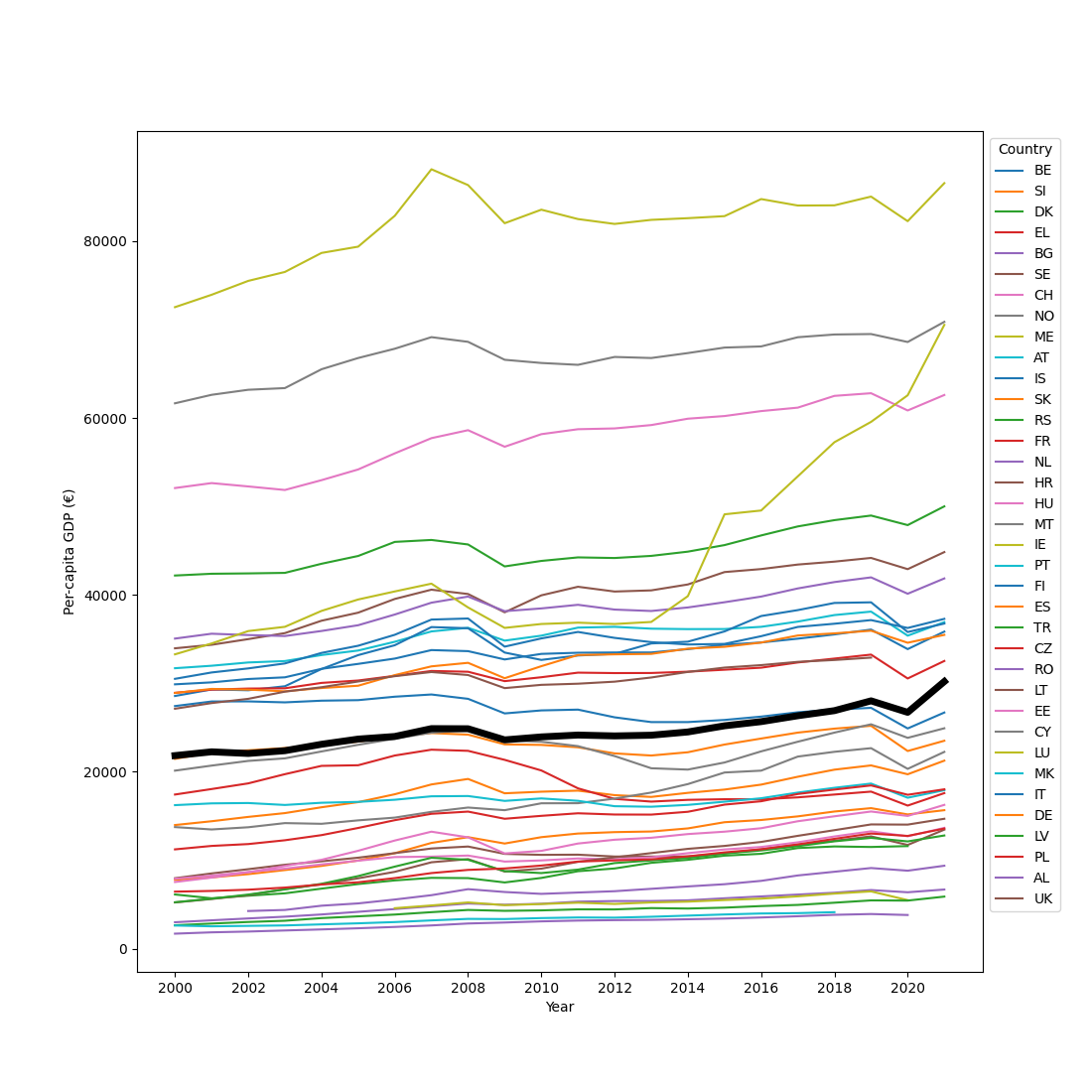### One-sample t-test

If we take the difference between the Moon and Other measurements of the above dataset, then we can test this difference against zero (or another value specified with the `popmean` keyword) with `ttest_1samp()`:

```from datamatrix import io
from scipy.stats import ttest_1samp

diff = dm.Moon - dm.Other
t, p = ttest_1samp(diff, popmean=0)
print('t = {:.4f}, p = {:.4f}'.format(t, p))
```

Output:

```t = 6.4518, p = 0.0000
```

## Regression

### Correlation / simple linear regression

This dataset, taken from Rotten Tomatoes, contains the 'freshness' rating and the Box Office profit for all of Adam Sandler's movies. You can use `linregress()` from `scipy.stats` to test if highly rated Adam Sandler movies make more money than poorly rated ones. (They don't.)

```from datamatrix import io
from scipy.stats import linregress

slope, intercept, r, p, se = linregress(dm.Freshness, dm['Box Office (\$M)'])
print('Box Office = {:.2f} * Freshness + {:.2f}'.format(slope, intercept))
print('p = {:.4f}, r = {:.4f}'.format(p, r))
```

Output:

```Box Office = -7.08 * Freshness + 80.13
p = 0.8785, r = -0.0286
```

To visualize this relationship, you can use Seaborn's `regplot()` function.

```from matplotlib import pyplot as plt
import seaborn as sns

sns.regplot(x='Freshness', y='Box Office (\$M)', data=dm)
plt.show()
```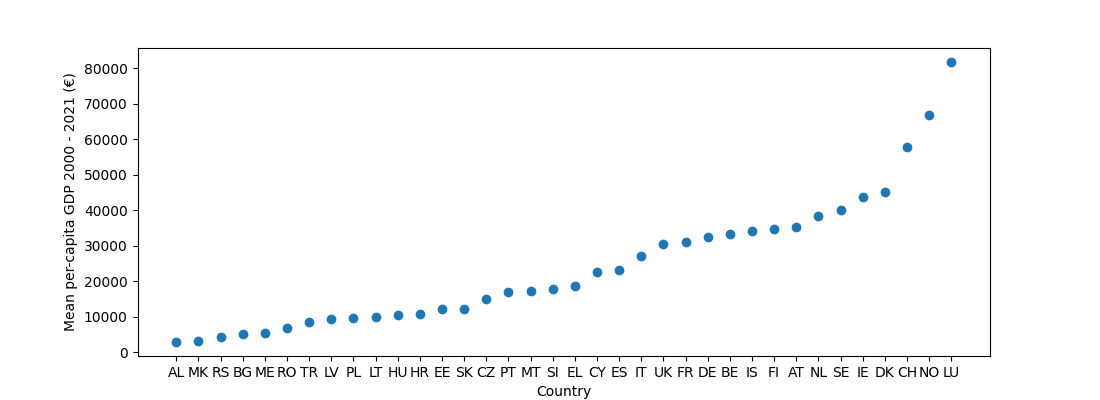### Multiple linear regression

Consider this dataset from Moore, McCabe, & Craig which contains grade-point averages (`gpa`) and SAT scores for mathematics (`satm`) and verbal knowledge (`satv`) for 500 high-school students. To test whether `satm` and `satv` are (uniquely) related to `gpa`, you can use the code below. (Only `satm` is uniquely related to `gpa`.)

The series of nested function calls (`ols(…).fit().summary()`) isn't very elegant, but the important part is the formula that is specified in a string with an R-style formula.

```from datamatrix import io
from statsmodels.formula.api import ols

print(ols('gpa ~ satm + satv', data=dm).fit().summary())
```

Output:

```                            OLS Regression Results
==============================================================================
Dep. Variable:                    gpa   R-squared:                       0.063
Method:                 Least Squares   F-statistic:                     7.476
Date:                Mon, 10 Aug 2020   Prob (F-statistic):           0.000722
Time:                        16:10:39   Log-Likelihood:                -254.18
No. Observations:                 224   AIC:                             514.4
Df Residuals:                     221   BIC:                             524.6
Df Model:                           2
Covariance Type:            nonrobust
==============================================================================
coef    std err          t      P>|t|      [0.025      0.975]
------------------------------------------------------------------------------
Intercept      1.2887      0.376      3.427      0.001       0.548       2.030
satm           0.0023      0.001      3.444      0.001       0.001       0.004
satv       -2.456e-05      0.001     -0.040      0.968      -0.001       0.001
==============================================================================
Omnibus:                       23.688   Durbin-Watson:                   1.715
Prob(Omnibus):                  0.000   Jarque-Bera (JB):               27.838
Skew:                          -0.809   Prob(JB):                     9.02e-07
Kurtosis:                       3.601   Cond. No.                     5.85e+03
==============================================================================

Warnings:
 Standard Errors assume that the covariance matrix of the errors is correctly specified.
 The condition number is large, 5.85e+03. This might indicate that there are
strong multicollinearity or other numerical problems.
```

## ANOVA

### ANOVA (regular)

Let's go back to this heart-rate data from Moore, McCabe, and Craig. This dataset contains two factors that vary between subjects (Gender and Group) and one dependent variable (Heart Rate). To test whether Gender, Group, or their interaction affect heart rate, you need the following code. (They all do.)

As above, the combination of `ols()` and `anova_lm()` isn't very elegant, but the important part is the formula.

```from datamatrix import io
from statsmodels.formula.api import ols
from statsmodels.stats.anova import anova_lm

dm.rename('Heart Rate', 'HeartRate')  # statsmodels doesn't like spaces
df = anova_lm(ols('HeartRate ~ Gender * Group', data=dm).fit())
print(df)
```

Output:

```                 df      sum_sq        mean_sq           F         PR(>F)
Gender          1.0   45030.005   45030.005000  185.979949   3.287945e-38
Group           1.0  168432.080  168432.080000  695.647040  1.149926e-110
Gender:Group    1.0    1794.005    1794.005000    7.409481   6.629953e-03
Residual      796.0  192729.830     242.122902         NaN            NaN
```

You can visualize this result with Seaborn:

```import seaborn as sns

sns.pointplot(x='Group', y='HeartRate', hue='Gender', data=dm)
plt.xlabel('Group')
plt.ylabel('Heart rate')
plt.show()
```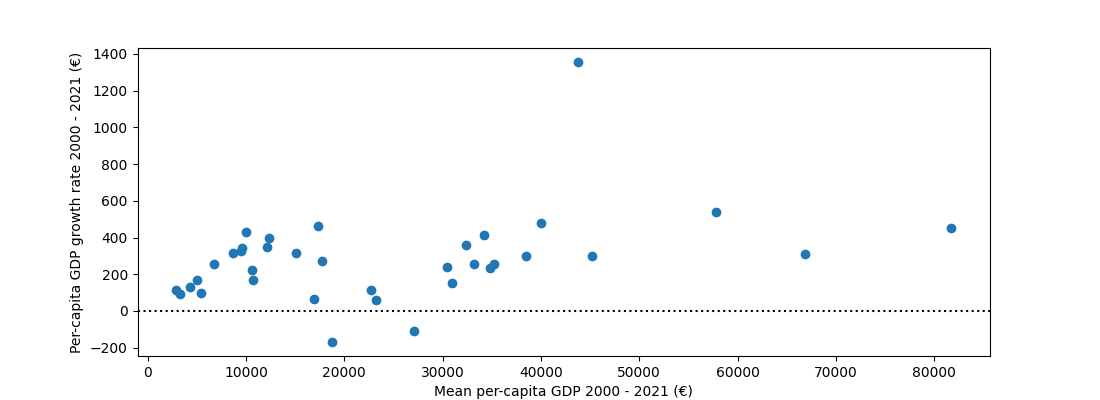### Repeated Measures ANOVA

A Repeated Measures ANOVA is generally used to analyze data from experiments in which all participants take part in all conditions, that is, a within-subject design. An example of such a design comes from an experiment by Zhou and colleagues, in which participants searched for a target object in the presence of a distractor object. Either the target, or the distractor, or both could match a color that participants held in memory. You can download this dataset here.

To test whether the factors distractor-match, target-match, and their interaction affect search accuracy, you can use the `AnovaRM` class from `statsmodels.stats.anova`. (They all do.)

Somewhat different most other RM-ANOVA software, the `AnovaRM` class accepts the data in long, unaggregated format. That is, each row corresponds to a single observation. Statsmodels will automatically reduce this format to a format where observations are aggregated per participant and condition (which is the required format for an RM-ANOVA) using the method indicated with the `aggregate_func` keyword:

```from pandas import pivot_table
from datamatrix import io
from statsmodels.stats.anova import AnovaRM

aov = AnovaRM(
dm,
depvar='search_correct',
subject='subject_nr',
within=['target_match', 'distractor_match'],
aggregate_func='mean'
).fit()
print(aov)
```

Output:

```                           Anova
===========================================================
F Value Num DF  Den DF Pr > F
-----------------------------------------------------------
target_match                   6.7339 1.0000 34.0000 0.0139
distractor_match              13.9729 1.0000 34.0000 0.0007
target_match:distractor_match  7.1687 1.0000 34.0000 0.0113
===========================================================
```

Let's visualize this result:

```import seaborn as sns

sns.pointplot(
x='target_match',
y='search_correct',
hue='distractor_match',
data=dm
)
plt.xlabel('Target match')
plt.ylabel('Search accuracy (proportion)')
plt.legend(title='Distractor match')
plt.show()
```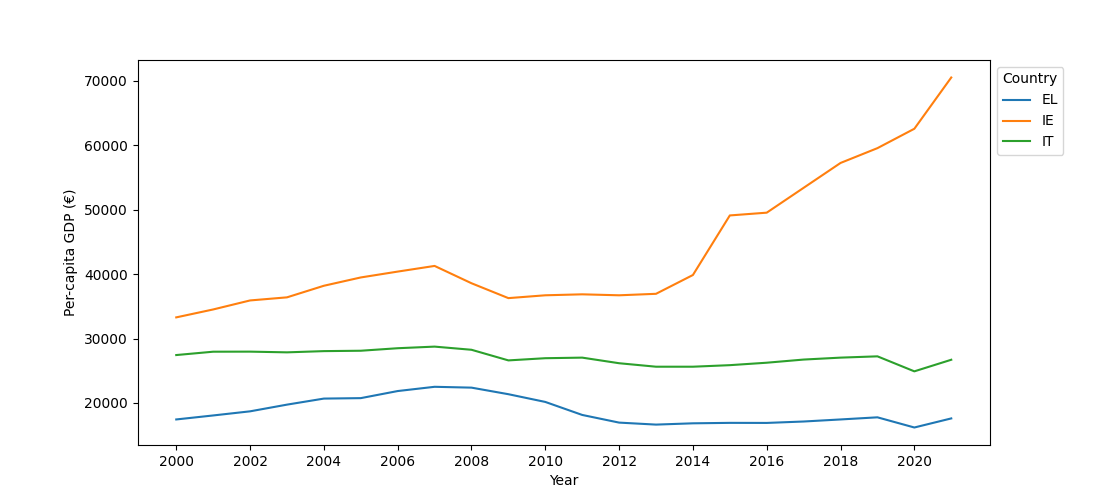Tip: If you prefer to conduct the RM-ANOVA with different software, such as JASP or SPSS, then you first need to create a so-called pivot table, in which each row corresponds to a subject, and each column to a condition. You can do this with the `pandas.pivot_table()` function:

```from pandas import pivot_table
from datamatrix import io

pm = pivot_table(
dm,
values='search_correct',
index='subject_nr',
columns=['target_match', 'distractor_match']
)
print(pm)
```

Output:

```target_match             0                   1
distractor_match         0         1         0         1
subject_nr
0                 0.968750  0.921875  1.000000  0.953125
1                 1.000000  0.968750  1.000000  0.937500
2                 1.000000  0.937500  1.000000  0.984375
3                 1.000000  0.953125  0.968750  0.968750
4                 0.921875  0.859375  1.000000  0.953125
5                 0.984375  0.968750  0.984375  1.000000
6                 1.000000  1.000000  1.000000  1.000000
7                 0.968750  0.968750  0.984375  1.000000
8                 1.000000  0.984375  1.000000  0.984375
9                 1.000000  0.984375  1.000000  1.000000
10                0.015625  0.031250  0.000000  0.000000
11                0.984375  0.937500  0.984375  0.953125
12                0.906250  0.843750  0.953125  0.953125
13                0.937500  0.984375  0.953125  0.968750
14                0.828125  0.812500  0.843750  0.812500
15                0.953125  0.921875  1.000000  0.984375
16                0.890625  0.843750  0.906250  0.890625
17                0.984375  0.968750  0.968750  0.968750
18                0.578125  0.484375  0.500000  0.515625
19                0.500000  0.562500  0.484375  0.562500
20                0.796875  0.812500  0.734375  0.796875
21                0.984375  0.906250  1.000000  0.937500
22                0.531250  0.468750  0.765625  0.671875
23                0.984375  0.968750  0.984375  0.984375
24                0.937500  0.921875  1.000000  0.953125
25                1.000000  0.968750  1.000000  0.968750
26                0.859375  0.812500  0.937500  0.921875
27                0.906250  0.921875  0.937500  0.890625
28                0.843750  0.796875  0.828125  0.828125
29                1.000000  0.968750  1.000000  1.000000
30                1.000000  0.984375  1.000000  0.984375
31                0.984375  0.953125  1.000000  0.968750
32                0.984375  0.906250  0.984375  0.906250
33                0.484375  0.484375  0.453125  0.515625
34                0.984375  0.921875  0.968750  0.984375
```

## Exercises

### A three-way Repeated Measures ANOVA

Above you have seen how to conduct a two-way repeated measures ANOVA with this dataset from Zhou et al. (2020). But the data contains a third factor: congruency. First, run a three-way repeated measures ANOVA with target-match, distractor-match, and congruency as independent variables, and search accuracy as dependent variable. Next, plot the results in a two-panel plot, where the left subplot shows the effect of distractor and target match for congruent trials, while the right subplot shows this for incongruent trials.

View solution

### Correlating activity in the left and right brain

• Read this dataset, which has been adapted from the StudyForrest project. See Exercise 2 from the NumPy tutorial if you don't remember this dataset!
• Get the mean BOLD response over time, separately for the left and the right brain.
• Plot the BOLD response over time for the left and the right brain.
• You will notice that there is a slight drift in the signal, such that the BOLD response globally increases over time. You can remove this with so-called detrending, using `scipy.signal.detrend()`.
• Plot the detrended BOLD response over time for the left and the right brain.
• Determine the correlation between the detrended BOLD response in the left and the right brain.

Statistical caveat: Measures that evolve over time, such as the BOLD response, are not independent. Therefore, statistical tests that assume independent observations are invalid for this kind of data! In this exercise, this means that the p-value for the correlation is not meaningful.

View solution